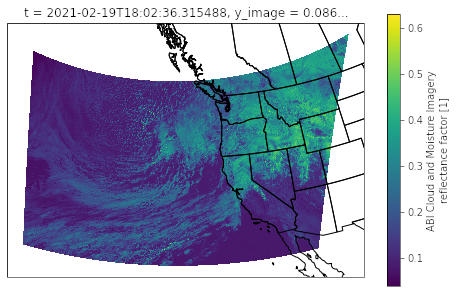# Let’s go!

`import xarray as xr import s3fsimport matplotlib.pyplot as pltimport numpy as npimport cartopy.crs as ccrsimport cartopy.feature as cfeature `
`fs = s3fs.S3FileSystem(anon=True)f = fs.open("s3://noaa-goes17/ABI-L2-MCMIPC/2021/050/18/OR_ABI-L2-MCMIPC-M6_G17_s20210501801176_e20210501803549_c20210501804089.nc")ds = xr.open_dataset(f) `
`def calc_latlon(ds):    # The math for this function was taken from     # https://makersportal.com/blog/2018/11/25/goes-r-satellite-latitude-and-longitude-grid-projection-algorithm    x = ds.x    y = ds.y    goes_imager_projection = ds.goes_imager_projection        x,y = np.meshgrid(x,y)        r_eq = goes_imager_projection.attrs["semi_major_axis"]    r_pol = goes_imager_projection.attrs["semi_minor_axis"]    l_0 = goes_imager_projection.attrs["longitude_of_projection_origin"] * (np.pi/180)    h_sat = goes_imager_projection.attrs["perspective_point_height"]    H = r_eq + h_sat        a = np.sin(x)**2 + (np.cos(x)**2 * (np.cos(y)**2 + (r_eq**2 / r_pol**2) * np.sin(y)**2))    b = -2 * H * np.cos(x) * np.cos(y)    c = H**2 - r_eq**2        r_s = (-b - np.sqrt(b**2 - 4*a*c))/(2*a)        s_x = r_s * np.cos(x) * np.cos(y)    s_y = -r_s * np.sin(x)    s_z = r_s * np.cos(x) * np.sin(y)        lat = np.arctan((r_eq**2 / r_pol**2) * (s_z / np.sqrt((H-s_x)**2 +s_y**2))) * (180/np.pi)    lon = (l_0 - np.arctan(s_y / (H-s_x))) * (180/np.pi)        ds = ds.assign_coords({        "lat":(["y","x"],lat),        "lon":(["y","x"],lon)    })    ds.lat.attrs["units"] = "degrees_north"    ds.lon.attrs["units"] = "degrees_east"return ds`
`def get_xy_from_latlon(ds, lats, lons):    lat1, lat2 = lats    lon1, lon2 = lons    lat = ds.lat.data    lon = ds.lon.data        x = ds.x.data    y = ds.y.data        x,y = np.meshgrid(x,y)        x = x[(lat >= lat1) & (lat <= lat2) & (lon >= lon1) & (lon <= lon2)]    y = y[(lat >= lat1) & (lat <= lat2) & (lon >= lon1) & (lon <= lon2)]         return ((min(x), max(x)), (min(y), max(y)))`
`ds = calc_latlon(ds)`
`lat                  (y, x) float64 53.5 53.49 53.49 ... 14.8 14.8 14.81lon                  (y, x) float64 -184.4 -184.3 -184.2 ... -112.5 -112.4`
`lats = (30, 55)lons = (-152, -112)`
`((x1,x2), (y1, y2)) = get_xy_from_latlon(ds, lats, lons)`
`subset = ds.sel(x=slice(x1, x2), y=slice(y2, y1))`
`p = subset.CMI_C01.plot(    x='lon', y='lat',     subplot_kws={'projection' : ccrs.Orthographic(-130, 40)},    transform = ccrs.PlateCarree())p.axes.add_feature(cfeature.COASTLINE)p.axes.add_feature(cfeature.STATES)`GOES blue channel plotted over the west coast of the United States
`# Get channels r = subset['CMI_C02'].data; r = np.clip(r, 0, 1)g = subset['CMI_C03'].data; g = np.clip(g, 0, 1)b = subset['CMI_C01'].data; b = np.clip(b, 0, 1)# Apply a gamma of 2.5gamma = 2.5; r = np.power(r, 1/gamma); g = np.power(g, 1/gamma); b = np.power(b, 1/gamma)# Calculate "true green" from the veggie channelg_true = 0.45 * r + 0.1 * g + 0.45 * bg_true = np.clip(g_true, 0, 1)rgb = np.dstack((r, g_true, b))`
`fig = plt.figure(figsize=(8,5))plt.imshow(rgb)`

--

--